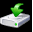# JavaScript : HTML Form validation - checking for password

Sometimes a password validation in a form is essential. You can create a password in different ways, it's structure may be simple, reasonable or strong. Here we validate various type of password structure through JavaScript codes and regular expression.

• Check a password between 7 to 16 characters which contain only characters, numeric digits and underscore and first character must be a letter.
• Check a password between 6 to 20 characters which contain at least one numeric digit, one uppercase and one lowercase letter.
• Check a password between 7 to 15 characters which contain at least one numeric digit and a special character.
• Check a password between 8 to 15 characters which contain at least one lowercase letter, one uppercase letter, one numeric digit, and one special character.

Following code blocks contain actual codes for the said validations. We have kept the CSS code part common for all the validations.

CSS Code:

``````li {list-style-type: none;
font-size: 16pt;
}
.mail {
margin: auto;
width: 400px;
background : #D8F1F8;
border: 1px soild silver;
}
.mail h2 {
margin-left: 38px;
}
input {
font-size: 20pt;
}
input:focus, textarea:focus{
background-color: lightyellow;
}
input submit {
font-size: 12pt;
}
.rq {
color: #FF0000;
font-size: 10pt;
}``````

## To check a password between 7 to 16 characters which contain only characters, numeric digits, underscore and first character must be a letter

To validate the said format we use the regular expression ^[A-Za-z]\w{7,15}\$, where \w matches any word character (alphanumeric) including the underscore (equivalent to [A-Za-z0-9_]).  Next the match() method of string object is used to match the said regular expression against the input value. Here is the complete web document.

HTML Code:

``````<!DOCTYPE html>
<html lang="en">
<meta charset="utf-8">
title>JavaScript form validation - Password Checking - 1</title>
<div class="mail">
<h2>Input Password and Submit [7 to 15 characters which contain only characters, numeric digits, underscore and first character must be a letter]</h2
<form name="form1" action="#">
<ul>
<li><input type='text' name='text1'/></li>
<li class="rq">*Enter numbers only.</li>
<li>&nbsp;</li>
<li class="submit"><input type="submit" name="submit" value="Submit" onclick="CheckPassword(document.form1.text1)"/></li>
<li>&nbsp;</li>
</ul>
</form>
</div>
</body>
</html>
``````

JavaScript Code:

``````function CheckPassword(inputtxt)
{
var passw=  /^[A-Za-z]\w{7,14}\$/;
if(inputtxt.value.match(passw))
{
return true;
}
else
{
return false;
}
}
``````

View the example in the browser

## To check a password between 6 to 20 characters which contain at least one numeric digit, one uppercase and one lowercase letter

To validate the said format we use the regular expression ^(?=.*\d)(?=.*[a-z])(?=.*[A-Z]).{4,8}\$.  Next the match() method of string object is used to match the said regular expression against the input value. Here is the complete web document.

HTML Code:

``````<!DOCTYPE html>
<html lang="en">
<meta charset="utf-8">
<title>JavaScript form validation - Password Checking - 2</title>
<div class="mail">
<h2>Input Password and Submit [6 to 20 characters which contain at least one numeric digit, one uppercase and one lowercase letter]</h2>
<form name="form1" action="#">
<ul>
<li><input type='text' name='text1'/></li>
<li class="rq">*Enter numbers only.</li>
<li>&nbsp;</li>
<li class="submit"><input type="submit" name="submit" value="Submit" onclick="CheckPassword(document.form1.text1)"/></li>
<li>&nbsp;</li>
</ul>
</form>
</div>
</body>
</html>``````

JavaScript Code:

``````function CheckPassword(inputtxt)
{
var passw = /^(?=.*\d)(?=.*[a-z])(?=.*[A-Z]).{6,20}\$/;
if(inputtxt.value.match(passw))
{
return true;
}
else
{
return false;
}
}
``````

View the example in the browser

## To check a password between 7 to 15 characters which contain at least one numeric digit and a special character

To validate the said format we use the regular expression ^^(?=.*[0-9])(?=.*[[email protected]#\$%^&*])[[email protected]#\$%^&*]{7,15}\$.  Next the match() method of string object is used to match the said regular expression against the input value. Here is the complete web document.

HTML Code:

``````<!DOCTYPE html>
<html lang="en">
<meta charset="utf-8">
<title>JavaScript form validation - Password Checking - 3</title>
<div class="mail">
<h2>Input Password and Submit [7 to 15 characters which contain at least one numeric digit and a special character]</h2>
<form name="form1" action="#">
<ul>
<li><input type='text' name='text1'/></li>
<li class="rq">*Enter numbers only.</li>
<li>&nbsp;</li>
<li><input type="submit" name="submit" value="Submit" onclick="allnumericplusminus(document.form1.text1)" /></li>
<li>&nbsp;</li>
</ul>
</form>
</div>
</body>
</html>
``````

JavaScript Code

``````function CheckPassword(inputtxt)
{
var paswd=  /^(?=.*[0-9])(?=.*[[email protected]#\$%^&*])[[email protected]#\$%^&*]{7,15}\$/;
if(inputtxt.value.match(paswd))
{
return true;
}
else
{
return false;
}
}
``````

View the example in the browser

## To check a password between 8 to 15 characters which contain at least one lowercase letter, one uppercase letter, one numeric digit, and one special character

To validate the said format we use the regular expression ^(?=.*\d)(?=.*[a-z])(?=.*[A-Z])(?=.*[^a-zA-Z0-9])(?!.*\s).{8,15}\$.  Next the match() method of string object is used to match the said regular expression against the input value. Here is the complete web document.

HTML Code:

``````<!DOCTYPE html>
<html lang="en">
<meta charset="utf-8">
<title>JavaScript form validation - Password Checking - 4</title>
<div class="mail">
<h2>Input Password and Submit [8 to 15 characters which contain at least one lowercase letter, one uppercase letter, one numeric digit, and one special character]</h2>
<form name="form1" action="#">
<ul>
<li><input type='text' name='text1'/></li>
<li class="rq">*Enter numbers only.</li>
<li>&nbsp;</li>
<li><input type="submit" name="submit" value="Submit" onclick="allnumericplusminus(document.form1.text1)" /></li>
<li>&nbsp;</li>
</ul>
</form>
</div>
</body>
</html>``````

JavaScript Code:

``````function CheckPassword(inputtxt)
{
var decimal=  /^(?=.*\d)(?=.*[a-z])(?=.*[A-Z])(?=.*[^a-zA-Z0-9])(?!.*\s).{8,15}\$/;
if(inputtxt.value.match(decimal))
{
return true;
}
else
{
return false;
}
}
``````

View the example in the browserDownload the Validation code from here
﻿

## JavaScript: Tips of the Day

|| operator

```const one = false || {} || null;
const two = null || false || '';
const three = [] || 0 || true;

console.log(one, two, three);
```

With the || operator, we can return the first truthy operand. If all values are falsy, the last operand gets returned.
(false || {} || null): the empty object {} is a truthy value. This is the first (and only) truthy value, which gets returned. one is equal to {}.
(null || false || ""): all operands are falsy values. This means that the last operand, "" gets returned. two is equal to "".
([] || 0 || ""): the empty array[] is a truthy value. This is the first truthy value, which gets returned. three is equal to [].

Ref: https://bit.ly/3jFRBje